Related Articles
Angle Sum Property of a Triangle
• Last Updated : 19 Jan, 2021

Lines that lie in the same plane and do not intersect are known as parallel lines. Parallel lines have equal distance between them. When a perpendicular line is drawn at some point on one line also intersects another line perpendicularly when extended.

Example:

The distance between points A and C is the same as the distance between points B and D.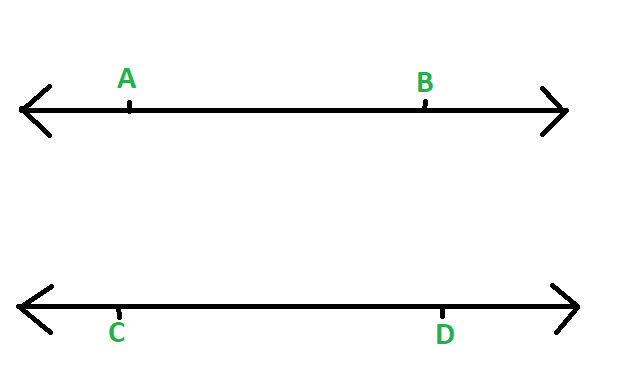Fig 1

### Theorem: Two or more lines that are parallel to the same line will be parallel to each other.

Proof:

Let us assume that a line ‘a’ is parallel to line ‘b’ and the distance between line a and b is constant that is ‘x’ and another set of the parallel line is line ‘b’ and line ‘c’ with a constant distance of ‘y’. We have to prove that two or more lines that are parallel to one another are parallel to each other so in this case, we need to prove that if line ‘a’ is parallel to line ‘b’ and if line ‘b’ is parallel to line ‘c’ then we need to prove that line ‘a’ is parallel to line ‘c’.

As we are given that the constant distance between the parallel line ‘a’ and ‘b’ is x and the distance between line ‘b’ and ‘c’ is y. So as shown in the figure we can see the distance between the line ‘a’ and ‘c’ is x + y = z. And as we know that x and y are constant so the sum of 2 constant is also a constant. And we have proved that line ‘a’ and ‘c’ are parallel to one another.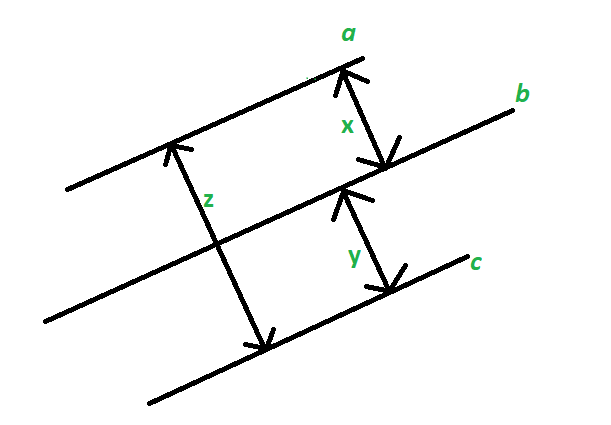Fig 2

### What is a transversal line?

Any line lying in the same plane of two or more lines and intersecting them at distinct points is known as a transversal line. According to Euclid’s parallel postulate, if the two lines are parallel, consecutive interior angles are supplementary that is they sum to 180 degrees, corresponding angles are equal, and alternate angles are equal.

Example:

Here line T is a transversal line.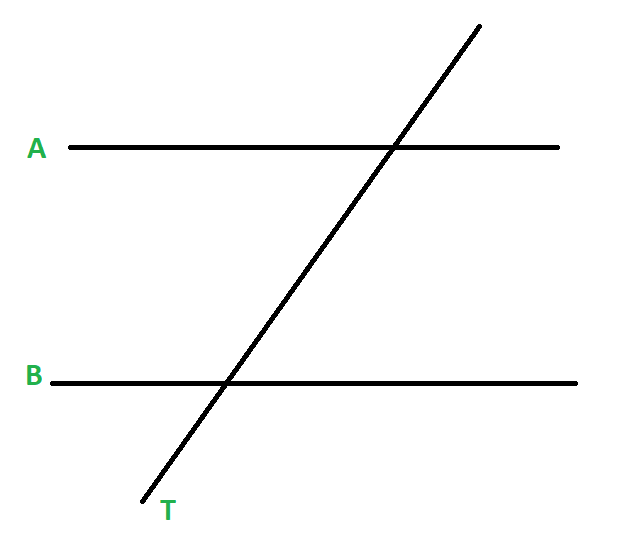Fig 3

### Angles formed by the intersection of Transversal line with Parallel Lines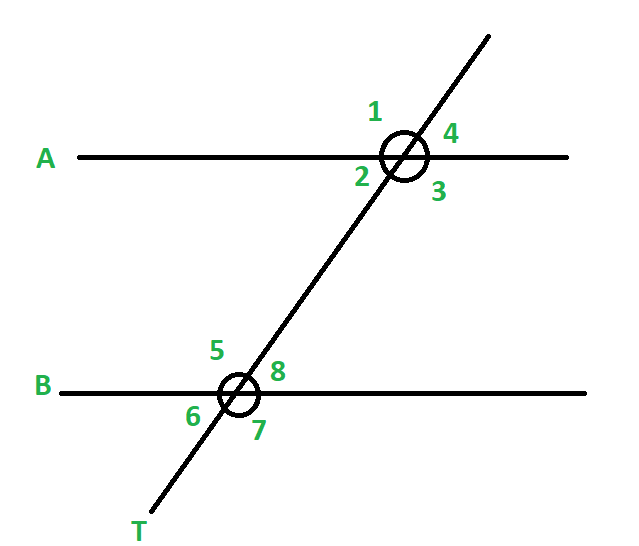Fig 4

Corresponding Angles

When two or more lines are cut by a transversal, then angles having the same relative position are called corresponding angles.

Example:

In the above Fig 4

∠1 and ∠5

∠2 and ∠6

∠3 and ∠7

∠4 and ∠8  are corresponding angles.

Consecutive Interior and Exterior Angles

When a line cuts parallel lines then angles that lie on the same side of the transversal line and inside the two parallel lines are called the consecutive interior angles.

Example:

In the above Fig 4

∠2 and ∠5

∠3 and ∠8  are consecutive interior angles.

The angles which lie on the same side of the transversal line and outside the two parallel lines are called the consecutive exterior angles.

Example:- In the above Fig 4

∠1 and ∠6

∠4 and ∠7  are consecutive exterior angles.

Supplementary Angles

Angles that add up to 180° are called supplementary angles. The pair of consecutive interior angles and consecutive exterior angles formed by 2 parallel lines are called supplementary angles.

Alternate Interior Angles

When a line cuts parallel lines then angles that lie on either side of the transversal line and inside the two parallel lines are called the alternate interior angles.

Example:

In the above Fig 4

∠2 and ∠8

∠3 and ∠5  are alternate interior angles.

Alternate Exterior Angles

When a line cuts parallel lines then angles that lie on either side of the transversal line and outside the two parallel lines are called the alternate exterior angles.

Example:

In the above Fig 4

∠1 and ∠7

∠4 and ∠6  are alternate exterior angles.

Congruent Angles

Angles that are of the same measure are called congruent angles. Corresponding and Alternate Angles are also congruent angles.

## Angle sum property of a triangle

### Theorem 1: The angle sum property of a triangle states that the sum of interior angles of a triangle is 180°.

Proof:

Sum of all the angles of a triangle is equal to 180° this theorem can be proved by the below-shown figure. When we draw a line parallel to any given side of a triangle let’s make a line AB parallel to side RQ of the triangle. In the given figure as we can see that side RP and sie QP act as a transversal. So we can see that angle ∠APR = ∠PRQ and ∠BPQ = ∠PQR by the property of alternate interior angles we have studied above. Therefore we can prove that

∠APR + ∠RPQ + ∠BPQ = 180° and Hence ∠PRQ + ∠RPQ + ∠PQR = 180°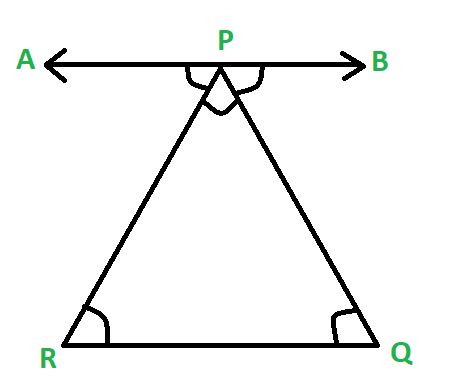Fig 5

Example: Finding angle measures using Angle Sum Property of a triangle if ∠PQR = 30°, ∠QRP = 70°?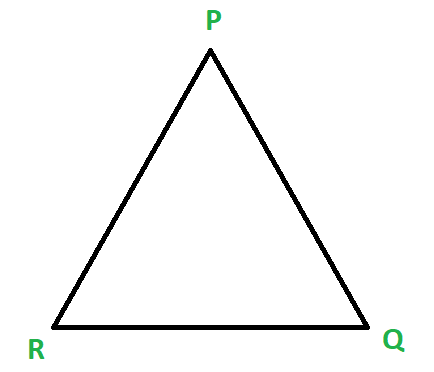Fig 6

Solution:

As we know that ∠PQR + ∠QRP + ∠RPQ = 180°.Threfore 30° + 70° + ∠RPQ = 180°.

=> 100° + ∠RPQ = 180°

=> ∠RPQ = 180° – 100°

=> ∠RPQ = 80°

### Theorem 2: If any side of a triangle is extended, then the exterior angle so formed is the sum of the two opposite interior angles of the triangle.

Proof:

As we have proved the sum of all the interior angles of a triangle is 180° (∠ACB + ∠ABC + ∠BAC = 180°) and we can also see in figure 7 that ∠ACB + ∠ACD = 180° due to the straight line. By the above two equations, we can conclude that

=> ∠ACD = 180° – ∠ACB

=> ∠ACD = 180° – (180° – ∠ABC – ∠CAB)

=> ∠ACD = ∠ABC + ∠CAB

Hence proved that If any side of a triangle is extended, then the exterior angle so formed is the sum of the two opposite interior angles of the triangle.

Example: A triangle has ∠BAC = 60° and ∠ABC = 60° find the measure of angle ∠ACB as shown in the figure below?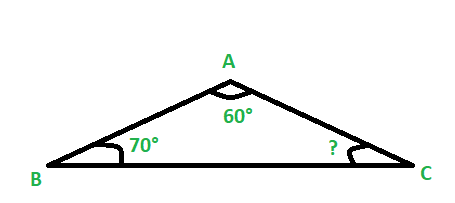Fig 7

Solution:

The solution to this problem can be approached in two ways:

Method 1:

By angle sum property of a triangle we know ∠ACB + ∠ABC + ∠BAC = 180°

So therefore ∠ACB = 180° – ∠ABC – ∠BAC

=> ∠ACB = 180° – 70° – 60°

=> ∠ACB = 50°

Method 2:

By exterior angle sum property of a triangle, we know that ∠ACD = ∠BAC + ∠ABC

=> ∠ACD = 70° + 60°

=> ∠ACD = 130°

and, ∠ACB = 180° – ∠ACD

=> ∠ACB = 180° – 130°

=> ∠ACB = 50°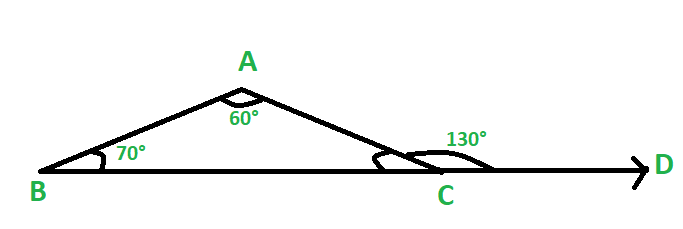Fig 8

Example: It is given that a transversal line cuts a pair of parallel lines and the ∠1 : ∠2 = 4 : 5 as shown in figure 9. Find the measure of the ∠3?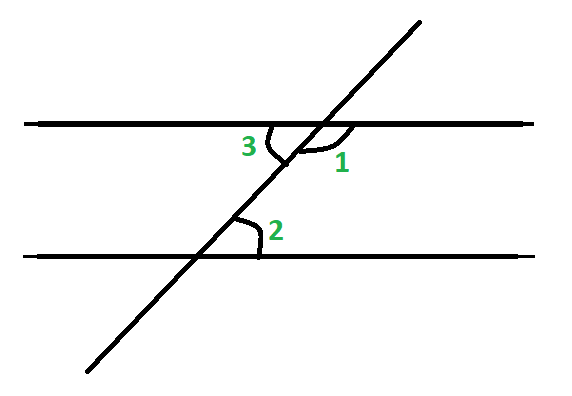Fig 9

Solution:

As we are given that the given pair of a line are parallel so we can see that ∠1 and ∠2 are consecutive interior angles and we have already studied that consecutive interior angles are supplementary. Therefore let us assume the measure of ∠1 as ‘4x’ therefore ∠2 would be ‘5x’ as we are given that  ∠1: ∠2 = 4: 5.

∠1 + ∠2 = 180°

=> 4x + 5x = 180°

=> 9x = 180°

=> x = 20°

Therefore ∠1 = 4x = 4 * 20° = 80° and ∠2 = 5x = 5 * 20° = 100°.

As we can clearly see in the figure that ∠3  and ∠2 are alternate interior angles so ∠3 = ∠2

∠3 = 100°.

Example: As shown in figure 10  angle APQ=120° and angle QRB=110°. Find the measure of the angle PQR given that the line AP is parallel to line RB?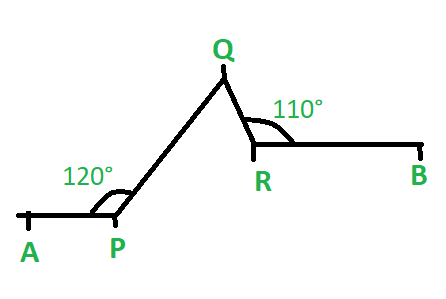Fig 10

Solution:

As we are given that line AP is parallel to line AP so we know that the line perpendicular to one would surely be perpendicular to the other. So let us make a line perpendicular to both the parallel line as shown in the picture. Now as we can clearly see that ∠APM + ∠MPQ = 120° and as PM is perpendicular to line AP so ∠APM = 90° therefore angle ∠MPQ = 120° – 90° = 30°. Similarly, we can see that ∠ORB = 90° as OR is perpendicular to line RB therefore ∠QRO = 110° – 90° = 20°. Line OR is parallel to line QN and MP therefore ∠PQN = ∠MPQ as they both are alternate interior angles and similarly ∠NQR = ∠ORQ. Therefore ∠PQR = ∠PQN + ∠NQR

=> ∠PQR = 30° + 20°

=> ∠PQR = 50°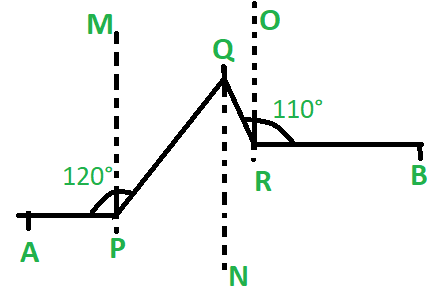Fig 11

My Personal Notes arrow_drop_up